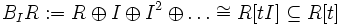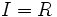Blowup algebra

Definition

Let$R$ be a commutative unital ring and$I$ be an ideal in$R$. The blowup algebra of$I$ in$R$ is defined as:$B_IR := R \oplus I \oplus I^2 \oplus \ldots \cong R[tI] \subseteq R[t]$

Note that$B_IR/IB_IR = gr_IR$, the associated graded ring to$I$ in$R$.

Particular cases

When$I$ is the zero ideal,$B_IR = R$. In other words,$R$ does not get blown up anywhere.

When$I = R$,$B_IR$ is the whole polynomial ring over$R$.